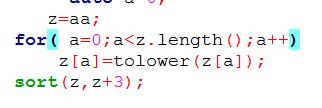###### Nieeee

2017-01-28 14:20 阅读 822

# 请教下为什么这种用法不对？sort 的用法报错
z 是 string 类型

• 点赞
• 写回答
• 关注问题
• 收藏
• 复制链接分享

#### 2条回答默认 最新

• 已采纳
`````` // sort algorithm example
#include <iostream>     // std::cout
#include <algorithm>    // std::sort
#include <vector>       // std::vector

bool myfunction (int i,int j) { return (i<j); }

struct myclass {
bool operator() (int i,int j) { return (i<j);}
} myobject;

int main () {
int myints[] = {32,71,12,45,26,80,53,33};
std::vector<int> myvector (myints, myints+8);               // 32 71 12 45 26 80 53 33

// using default comparison (operator <):
std::sort (myvector.begin(), myvector.begin()+4);           //(12 32 45 71)26 80 53 33

// using function as comp
std::sort (myvector.begin()+4, myvector.end(), myfunction); // 12 32 45 71(26 33 53 80)

// using object as comp
std::sort (myvector.begin(), myvector.end(), myobject);     //(12 26 32 33 45 53 71 80)

// print out content:
std::cout << "myvector contains:";
for (std::vector<int>::iterator it=myvector.begin(); it!=myvector.end(); ++it)
std::cout << ' ' << *it;
std::cout << '\n';

return
``````
点赞 1 评论 复制链接分享
• 这是java么，java的字符串不能按照下标索引，可以先调用toCharArray得到一个字符数组

点赞 2 评论 复制链接分享